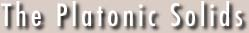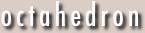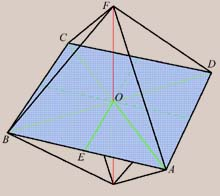Last revision Wednesday 28 August 1996Octahedron (f = 8, e = 12, v = 6)  Like the cube, the octahedron's ex- and inradius are very easy to determine. As usual, we let the side of the octahedron have length 1. Thus AE = 1/2. Now, it is clear that ABCD is a square (from symmetry). Then O is the center of this square, and is the foot of the perpendicular dropped from vertex F. Thus OE = EA, and OA = OB = OF. So OA = Sqrt/2 = 1/Sqrt, which is the exradius Ro. To find the inradius, not shown here, we construct the perpendicular from point O to the center of equilateral triangle ABF at G. AGO being right, GO2 + AG2 = OA2. Since AG2 = EG2 + EA2 = 4/3 EA2, ro2 = GO2 = OA2 - 4/3 EA2 = 1/2 - (4/3)(1/4) = 1/6, and ro = 1/Sqrt.